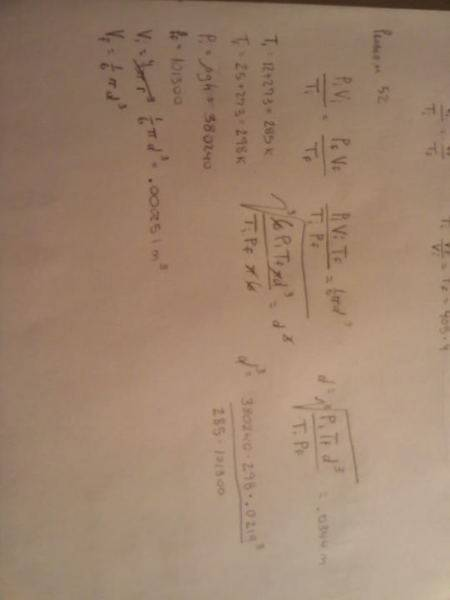# A diver and an air bubble

## Homework Statement

A diver 38.8 m deep in 14°C fresh water exhales a 2.19 cm diameter bubble. What is the bubble's diameter just as it reaches the surface of the lake, where the water temperature is 25°C?

PV=nRT

## The Attempt at a Solution

I dont know where to start on this problem at all I can find the intial pressure of the bubble but I dont see how that matters?

cepheid
Staff Emeritus
Gold Member
Helium balloons expand as they rise in the atmosphere, because the air gets thinner (and the air pressure reduces as a result). The same thing happens here. The pressure falls with rising height. So you can calculate the pressure at the two depths and see how it changes. However, the temperature also changes. As you know, from the ideal gas law, both of these things affect the volume.

I didn't think about that way. Let me work through it and then post what I got, thanks

What would be the initial pressure be? I know the final would be sea level of 101300 Pa.

I found Pi should be 3.38 atm x 101300 Pa= 393044 Pa because since he is 38.8 meters below sea level the pressure is 3.38 atm

cepheid
Staff Emeritus
Gold Member
Yeah, I guess the pressure at the surface would be be atmospheric pressure. Do you know how the pressure varies with depth in a fluid?

i approximated it that ever 10 meters below sea level= 1 atm is there a way to solve it for another way?

cepheid
Staff Emeritus
Gold Member
because since he is 38.8 meters below sea level the pressure is 3.38 atm

How do you figure that?

cepheid
Staff Emeritus
Gold Member
i approximated it that ever 10 meters below sea level= 1 atm is there a way to solve it for another way?

Interesting. Where did you find this approximation? It turns out to be more or less correct (for water). However, it might be helpful for you to know where it came from. Have you seen this before?

http://hyperphysics.phy-astr.gsu.edu/hbase/pflu.html#fp

Now you know the principle behind it, so you can figure it out precisely (it's not exactly 1 atm per 10 m) and you can also do this for any fluid.

I am still wrong here is my work....

cepheid
Staff Emeritus
Gold Member
By the way, 38.8 m / 10 m = 3.88, not 3.38 as you have written.

it is attached

#### Attachments

•2physics.jpg
9.7 KB · Views: 543
cepheid
Staff Emeritus
Gold Member
Your attachment won't be approved right away (I can't see the image). If you want immediate help, use a free online image hosting service to post the image, or type up your work.

PiVi/Ti=PfVf/Tf solved for Vf

Vf=PiViTf/TiPf then plugged in 1/6d^3 for the volumes and then found out that.....

df^3=PiTfdi^3/TiPf.......(380240*298*.0219^3/(285*101300))^1/3 and then got .125 m

cepheid
Staff Emeritus
Gold Member
The volume of a sphere is given by (4/3)πr3

Even converting to diameter, that turns into (π/6)d3, which is not the same as what you have written there.

I haven't checked your other work closely yet.

Oh right, but it cancels either waythat is the work i did before i switched the pressure so the anwser i really got is not .0344 m( either way thats wrong too) but .1235 m

cepheid
Staff Emeritus
Gold Member
The initial temperature is 287 K, not 285 K.

I should have an answer soon, and then we can compare.

ok thank you

cepheid
Staff Emeritus
Gold Member
I'm not gonna reveal my answer yet ;)

Here's what I have:

$$V_f = \frac{P_i}{P_f}\frac{T_f}{T_i}V_i$$

$$\frac{\pi}{6}d_f^3 = 3.88\frac{298}{287} \frac{\pi}{6}d_i^3$$

$$d_f = \sqrt{3.88\frac{298}{287}d_i^3}$$​

Is it any different from your work so far?

EDIT: I see you have exactly the same thing as I do. So, do you get the right answer with the corrected numbers?

nope it is the same except i changed my pressures to Pa instead of leaving it in atms like you.

cepheid
Staff Emeritus
Gold Member
nope it is the same except i changed my pressures to Pa instead of leaving it in atms like you.

Yeah that's a good point. If I don't use the approximation, I get that the ratio of pressures should be closer to 3.76 rather than 3.88.

Also, your initial pressure seems a bit off.

And doesn't 1 atm = 101 325 Pa?

What is the answer *supposed* to be?

I dont know it is online. It just told me my anwser is wrong.

I am thinking that there is something wrong with his C++ language in the program.